## External Memory Data Format

To load the input image to the deployed deep learning processor IP core and retrieve the output results, you can read data from the external memory and write data to the external memory by using the `dlhdl.Workflow` workflow. This workflow formats your data. Or, you can manually format your input data. Process the formatted output data by using the external memory data format.

### Key Terminology

• `Parallel Data Transfer Number` refers to the number of pixels that are transferred every clock cycle through the AXI master interface. Use the letter `N` in place of the ```Parallel Data Transfer Number```. Mathematically N is calculated as `power(2,nextpow2(sqrt(ConvThreadNumber)))`. For example, if the convolution thread number is nine, the calculated value of N is four. See ConvThreadNumber.

• `Feature Number` refers to the value of the z dimension of an x-by-y-by-z matrix. For example, most input images are of dimension x-by-y-by-three, with three referring to the red, green, and blue channels of an image. Use the letter `Z` in place of the `Feature Number`.

• `Thread Number` refers to the number of channels of the input that are operated upon simultaneously in a convolution style layer. Use the letter `C` in place of the `Thread Number`. Mathematically `C` is calculated as `sqrt(ConvThreadNumber)`. For example, if the convolution thread number is nine, the calculated value of `C` is three. See ConvThreadNumber.

### Convolution Module External Memory Input Data Format

The `single` data type inputs of the deep learning processor convolution module are typically three-dimensional (3-D).The external memory stores the data in a one-dimensional (1-D) vector. Converting the 3-D input image into 1-D to store in the external memory :

1. Sends `N` number of data in the `z` dimension of the matrix.

2. Sends the image information along the x dimension of the input image.

3. Sends the image information along the y dimension of the input image.

4. After the first `NXY` block is completed, we then send the next `NXY` block along the `z` dimension of the matrix.

The image demonstrates how the data stored in a 3-by-3-by-4 matrix is translated into a 1-by-36 matrix that is then stored in the external memory.When the image `Feature Number` (Z) is not a multiple of the `Parallel Data Transfer Number` (N), then we must pad a zeroes matrix of size x-by-y along the z dimension of the matrix to make the image `Z` value a multiple of `N`.

For example, if your input image is an x-by-y matrix with a `Z` value of three and the value of `N` is four, pad the image with a zeros matrix of size x-by-y to make the input to the external memory an x-by-y-by-4 matrix.

This image is the input image format before padding.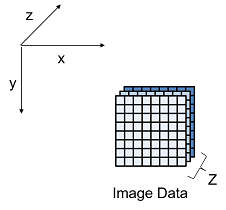This image is the input image format after zero padding.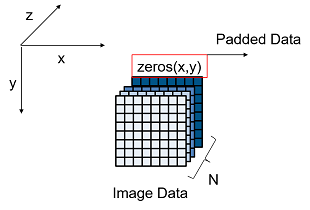The image shows the example output external memory data format for the input matrix after the zero padding. In the image, A, B, and C are the three features of the input image and G is the zero- padded data to make the input image `Z` value four, which is a multiple of `N`.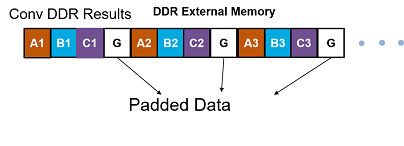If your deep learning processor consists of only a convolution (conv) processing module, the output external data is using the conv module external data format, which means it possibly contains padded data if your output `Z` value is not a multiple of the `N` value. The padded data is removed when you use the `dlhdl.Workflow` workflow. If you do not use the `dlhdl.Workflow` workflow and directly read the output from the external memory, remove the padded data.

When the `Thread Number` `C` is not a power of two and lower than N, then we must pad a zeroes matrix of size x-by-y along the z dimension of the matrix. The zeroes matrix is inserted after every C number of elements along the z dimension of the matrix to make the Z value a multiple of N.

For example, if your input image is an x-by-y matrix with a `C` value of three and `N` and `Z` values of four, pad the image with a zeroes matrix of size x-by-y after the third channel and three zeroes matrices of x-by-y after the fourth channel to make the input to the external memory an x-by-y-by-eight matrix.

This image is the input image format before padding.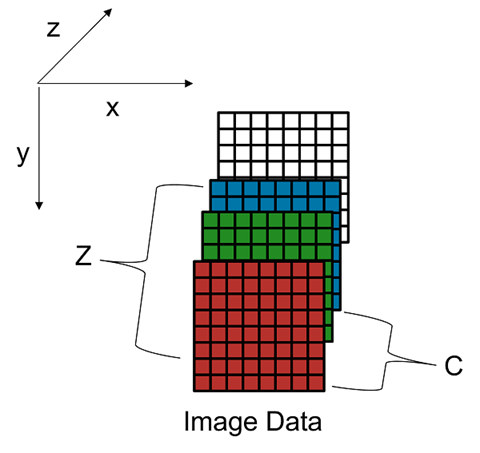This image is the input image format after zero padding.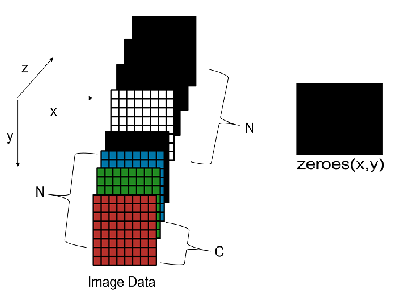This image shows a sample three-by-three-by-four matrix passed as an input to a deep learning processor configuration with a `C` value of three and `N` value of four.The image shows the example output external memory data format for the input matrix after the zero padding.When the values of `C` and `N` are equal, padding is required only when `Z` is not a multiple of `C`.

#### Convolution Module External Memory Output Data Format

The `single` data type outputs of the deep learning processor convolution module are typically three-dimensional (3-D).The external memory stores the data in a one-dimensional (1-D) vector. The external memory uses four bytes for a value. If the `Thread Number` is four, then four single values are concatenated into a 128-bit wide vector and stored in the external memory. When the `single` data type is stored in the memory the addresses are incremented in steps of four.

This example describes how the padded input data for a 3-by-3-by-5 matrix with `Thread Number` four is recovered from a 1-D vector in the external DDR memory. The original input size is a 3-by-3-by-5 matrix and the ```Thread Number``` is four. To make the third dimension a multiple of four, three 3-by-3-by-3 matrices are padded to make the padded input to the external memory a 3-by-3-by-8 matrix. The 3-by-3-by-8 matrix is converted into a 1-by-72 vector in the external memory. To convert the 1-D memory data back into a 3-D output matrix:

1. Sends `C` number of data in the `z` dimension of the matrix.

2. Sends the image information along the x dimension of the input image.

3. Sends the image information along the y dimension of the input image.

4. After the first `CXY` block is completed, we then send the next `CXY` block along the `z` dimension of the matrix.

This image demonstrates how the data in a 1-by-72 vector in memory is translated into a 3-by-3-by-8 matrix as the convolution module output.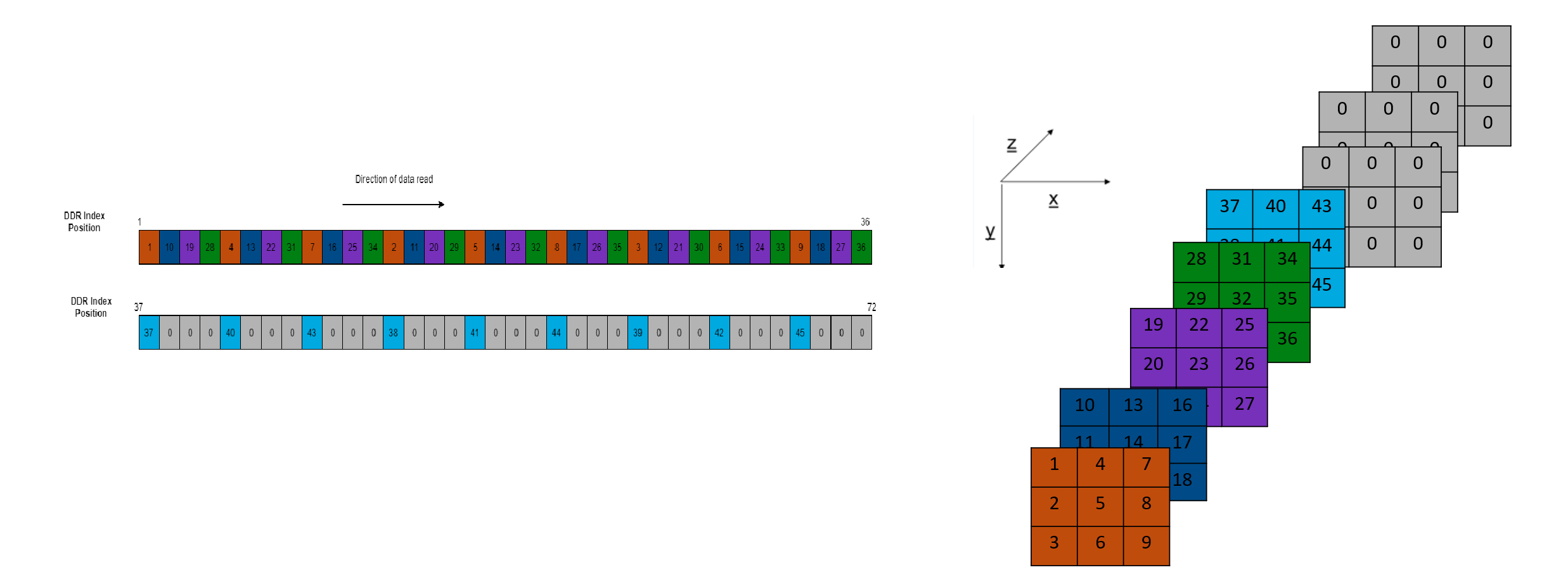As part of the post-processing, remove the three 3-by-3 matrices with zero data and return the output 3-by-3-by-5 matrix.

`int8` Data Type External Memory Format.  The `int8` data type outputs of the deep learning processor convolution module are typically three-dimensional (3-D).The external memory stores the data in a one-dimensional (1-D) vector. The external memory uses one byte for a value. If the `Thread Number` is four, then one byte per thread is concatenated into a 32-bit wide vector and stored in the external memory. When the `int8` data type is stored in the memory the addresses are incremented in steps of one.

#### Calculation of Output Memory Size

The size of the output for a deep learning processor IP core depends on the `Feature Number(Z)`, `Thread Number (C)`, and the `Parallel Data Thread Number (N)`. The formula to calculate the output memory size is `dimension1 * dimension2 * ceil(Z/C) * N`. For example, for an input matrix of size three-by-three-by-four the output memory size for a `C` and `N` value of four is ```3 *3 *ceil(4/4) *4 = 36```. In this example the output is written four values at a time because the value of `N` is four.

For a three-by-three-by-four matrix with a `C` value of three and `N` value of four, the output size is ```3 *3 *ceil(4/3) *4 =72```. In this example even when the output is written four values at a time only the first three values are valid as the fourth value is a zero padded value.

### Fully Connected Module External Memory Data Format

If your deep learning network consists of both the convolution (conv) and fully connected (fc) layers, the output of the deep learning (DL) processor follows the fc module external memory data format.

The image shows the example external memory output data format for a fully connected output feature size of six. In the image, A, B, C, D, E, and F are the output features of the image.